Courses

# Important Questions (1 Mark): Polynomials

## 25 Questions MCQ Test Mathematics (Maths) Class 10 | Important Questions (1 Mark): Polynomials

Description
This mock test of Important Questions (1 Mark): Polynomials for UPSC helps you for every UPSC entrance exam. This contains 25 Multiple Choice Questions for UPSC Important Questions (1 Mark): Polynomials (mcq) to study with solutions a complete question bank. The solved questions answers in this Important Questions (1 Mark): Polynomials quiz give you a good mix of easy questions and tough questions. UPSC students definitely take this Important Questions (1 Mark): Polynomials exercise for a better result in the exam. You can find other Important Questions (1 Mark): Polynomials extra questions, long questions & short questions for UPSC on EduRev as well by searching above.
QUESTION: 1

### Form a quadratic polynomial, sum of whose zeroes is 3 and product of whose zeroes is 2 solution

Solution:

Sum of zeros = 3/1
-b/a = 3/1
Product of zeros = -2/1
c/a = -2/1
This gives
a = 1
b = -3
c = -2,
The required quadratic equation is
ax2+bx+c
So,  x2-3x-2

QUESTION: 2

### If x + 2 is a factor of x3 – 2ax2 + 16, then value of a is

Solution:

Given ,g(x)=x+2=0
x=-2

P(x)=x3-2ax2+16
p(-2)=(-23)-2×a×(-22)+16
= -8 - 8a +16
= 8 - 8a
⇒ -8a = -8
⇒ a=1
Hence, value of a=1

So option B is correct answer.

QUESTION: 3

### If 3 + 5 – 8 = 0, then the value of (3)3 + (5)3 – (8)3 is

Solution:

Given :
3 + 5 + (-8) = 0.

Then,
3³ + 5³ + (-8)³ = ?

Using, identity...
If a+b+c=0, then a³ + b ³ + c³ = 3abc

Let a = 3, b = 5 and c = -8.

Here, a + b + c = 3 + 5 + (-8) = 0.

Then, a³ + b³ + c³ = 3³ + 5³ + (-8)³
= 3× 3 × 5 × -8
= 9 × -40
= -360

QUESTION: 4

If one of the factors of x2 + x – 20 is (x + 5), then other factor is

Solution:

Using mid-term splitting,
x2+x-20=x2+5x-4x-20=x(x+5)-4(x+5)
Taking common x+5
(x+5)(x-4) , so the other factor is x-4

QUESTION: 5

If α,β be the zeros of the quadratic polynomial 2x2 + 5x + 1, then value of α + β + αβ =

Solution:

P(x) = 2x² + 5x + 1
Sum of roots = -5/2
Product of roots = 1/2
Therefore substituting these values,
α + β +αβ
=(α + β) + αβ
= -5/2 + 1/2
= -4/2
= -2

QUESTION: 6

If α,β be the zeros of the quadratic polynomial 2 – 3x – x2, then α + β =

Solution:

We have Sum of zeros = -b/a
+=-b/a=-(-3)/(-1)=-3
Which is none of the above.

QUESTION: 7

Quadratic polynomial having sum of it's zeros 5 and product of it's zeros – 14 is –

Solution:

The quadratic equation is of the form  x- (sum of zeros) x + (product of zeros)
=x- 5x - 14

QUESTION: 8

If x = 2 and x = 3 are zeros of the quadratic polynomial x2 + ax + b, the values of a and b respectively are :

Solution:

Zeros of the polynomials are the values which gives zero when their value is substituted in the polynomial
When x=2,
x2+ax+b =(2)2+a*2+b=0
4+2a+b=0
b=-4-2a    ….1
When x=3,
(3)2+ 3a + b=0
9 + 3a + b=0
Substituting
9 + 3a - 4 - 2a =0
5 + a =0
a = -5
b = 6

QUESTION: 9

If 3 is a zero of the polynomial f(x) = x4 – x3 – 8x2 + kx + 12, then the value of k is –

Solution:
QUESTION: 10

The sum and product of zeros of the quadratic polynomial are – 5 and 3 respectively the quadratic polynomial is equal to –

Solution:
QUESTION: 11

On dividing x3 – 3x2 + x + 2 by polynomial g(x), the quotient and remainder were x – 2 and 4 – 2x respectively then g(x) :

Solution:

We have remainder theorem as
x3-3x2+x+2=q(x)*g(x)+r(x)
x3-3x2+x+2=(x-2)g(x)+(4-2x)
g(x)=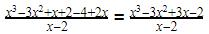So by division method,
g(x)= x- x + 1

QUESTION: 12

If the polynomial 3x2 – x3 – 3x + 5 is divided by another polynomial x – 1 – x2, the remainder comes out to be 3, then quotient polynomial is –

Solution:
QUESTION: 13

If x + 2 is a factor of x3 – 2ax2 + 16, then value of a is

Solution:
QUESTION: 14

If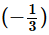is the zero of the cubic polynomial f(x) = 3x3 – 5x2 – 11x – 3 the other zeros are :

Solution:
QUESTION: 15

If p and q are the zeroes of the polynomial x2- 5x - k. Such that p - q = 1, find the value of K

Solution:
QUESTION: 16

Let p(x) = ax2 + bx + c be a quadratic polynomial. It can have at most –

Solution:
QUESTION: 17

The graph of the quadratic polynomial ax2 + bx + c, a ≡ 0 is always –

Solution:

We have a=0 So,
ax+ bx + c = 0*x+ bx + c = bx + c
Which is a linear equation in one variable
Linear equation as the name suggests is an equation which when plotted gives a straight line.

QUESTION: 18

If 2 and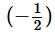as the sum and product of its zeros respectively then the quadratic polynomial f(x) is –

Solution:
QUESTION: 19

If α and β are the zeros of the polynomial f(x) = 16x2 + 4x – 5 then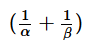is equal to –

Solution:
QUESTION: 20

If α and β are the zeros of the polynomial f(x) = 15x2 – 5x + 6 then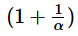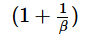is equal to –

Solution:

The correct answer is a.

(1+1/α) (1+1/β)

= (α+1/α) (β+1/β)

=αβ + α + β + 1 / αβ

=( 2/5 + 1/3+ 1)  /  2/5

=26/15 X 5/2 = 13/3

QUESTION: 21

If the sum of the two zeros of x3 + px2 +qx + r is zero, then pq =

Solution:

Sum of two solutions is zero ,so both are inverse of each other. So we have the solutions as a,-a,b.
(x-a)(x+a)(x+b)=(x2-a2)(x+b)=x3+bx2-a2x-a2b
pq=-a2b=r

QUESTION: 22

Let a = 0 and p(x) be a polynomial of degree greater than 2. If p(x) leaves remainders a and – a when divided respectively by x + a and x – a, the remainder when p(x) is divided by x2 – ais

Solution:

Let P(x)=(x−a)(x+a)Q(x)+rx+s
When we divide by x+a, we get
P(−a)=(−a−a)(−a+a)Q(−a)+r(−a)+s=a
⇒s−ra=a   ...(1)
And when we divide by x−a, we get
P(a)=(a−a)(a+a)Q(a)+ra+s=−a
⇒s+ra=−a    ...(2)
Solving (1) and (2), we get
s=0,r=−1
Hence, P(x)=(x−a)(x+a)Q(x)−x
And when we divide by x2-a2, it leaves a remainder −x.

QUESTION: 23

If one root of the polynomial x2 + px + q is square of the other root, then –

Solution:

Let one root be a, other root is a2.
(x-a)(x-a2)=x2+(-a2-a)x+a3
p3=(-a2-a)3=-a6-3a5-3a4-a3
-q(3p-1)= -a3(3(-a2-a)-1)=3a5+3a4+a3
q2=a6
Substituting the values
-a6-3a5-3a4-a3+3a5+3a4+a3+a6=0

QUESTION: 24

If α,β are the zeros of x2 + px + 1 and γ,δ be those of x2 + qx + 1, then the value of (α–γ) (β–γ) (α+δ) (β+δ) =

Solution:

Alpha(a) and beta(b) are roots of x^2 + px + 1

This implies that sum of roots= a+b = -p/1=-p

And the product of roots = ab = 1/1=1

Similarly ,

Gamma(c) and delta(d) are roots of x^2 + qx + 1

So c+d=-q and cd =1.

The above results can be obtained once we know that any quadratic equation has two roots and hence can be written as (x-p)(x-q)=0 where a and b are the roots .

So x^2 -(p+q)x + pq =0

Comparing this with the general form of quadratic equation :ax^2 + bx + c= 0 we get

Sum of the roots =p+q= -b/a

And product of the roots = pq = c/a}

RHS=(a-c)(b-c)(a+d)(b+d)

=(c^2-ac-bc +ab)(d^2 +bd +ad + ab)

We know ab=1

So RHS= (c^2-ac-bc +1)(d^2 +bd +ad + 1)

= (c^2)(d^2) +(a+b)c^2(d) + c^2 -(d^2)c(a+b) -(a+b)^2(cd) -(a+b)c + d^2 + bd + ad + 1

= 1 + ac+bc + c^2 - da-db - (a^2 + b^2 + 2(1)) -ac -bc + bd + ad +1

Cancelling off all the common terms,

We get c^2 +d^2-a^2-b^2

= c^2+d^2-a^2-b^2
=c^2 -a^2 + d^2 -b^2 + 2(1) -2(1){ ab=cd =1}
=c^2 + d^2 + 2cd - a^2 - b^2 - 2ab
LHS=(c+d)^2-(a+b)^2
Therefore,
But we know -p= a+b and -q = c+d
LHS= q^2-p^2

QUESTION: 25

The quadratic polynomial whose zeros are twice the zeros of 2x2 – 5x + 2 = 0 is –

Solution:

Let α and β be the roots of the given equation.
Then, α + β = 5/2 and αβ = 2/2 = 1
∴ 2α + 2β
∴ 2(α + β)
∴ 5,(2α)(2β) = 4
So, the requiared equation is :
x2−5x+4=0